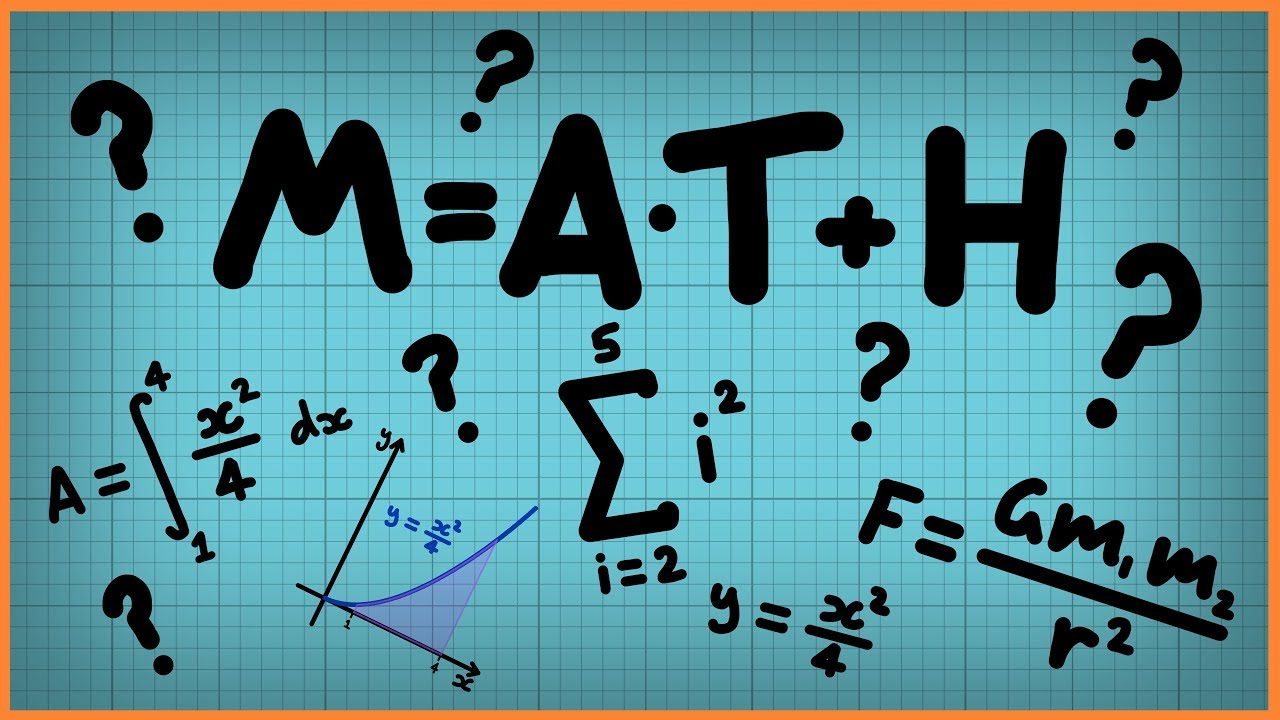Qalaxia Master Bot
0

|| 2.8 || Absolute errors || Relative Errors || Percentage Errors || Class ...

May 5, 2018 ... Absolute errors, Relative Errors, Percentage Errors, Class 11 Physics, Physics, Theory of errors, Units & Measurements,

For more information, see || 2.8 || Absolute errors || Relative Errors || Percentage Errors || Class ...Guru Nanak
0

Required formulas:

Arithmetic mean \mu = \frac{\Sigma_{1}^{n} a_i}{n}

The absolute error of measurement is the magnitude of the difference between the individual measurement and the true value(mean) of the quantity.

Absolute error , \Delta T = a_1 - \mu

The relative error is the ratio of the mean absolute error to the quantity measured's mean value.

\delta a = \frac{\text{ mean of } \Delta a }{\mu}

When the relative error is expressed as a percentage, it is referred to as the percentage error.

\delta a=\frac{\text{ mean of }\Delta a}{\mu}*100

Step 1: Find the mean of the oscillation reading of a pendulum.

Given that

Readings of the duration of oscillations of a pendulum

a_1 = 2.63 s, a_2 = 2.56 s, a_3 = 2.42 s, a_4 = 2.71s \text{ and } a_5 = 2.80 s.

Mean \mu = \frac{2.63 + 2.56 + 2.42 + 2.71 + 2.80}{5}

\mu = \frac{13.12}{5} = 2.624 = 2.62 seconds

Step 2: Determine the absolute error of measurements

Absolute error ( \Delta a )

\Delta a_1 = a_1 - \mu = 2.63 - 2.62 = 0.01 seconds

\Delta a_2 = a_2 - \mu = 2.56 - 2.62 = 0.06 seconds

\Delta a_3 = a_3 - \mu = 2.42 - 2.62 = 0.20 seconds

\Delta a_4 = a_4 - \mu = 2.71 - 2.62 = 0.09 seconds

\Delta a_5 = a_5 - \mu = 2.80 - 2.62 = 0.18 seconds

Step 3: Identify the relative error and convert it into percentage error

Relative error, \delta a = \frac{\text{ mean of } \Delta a }{\mu}

\Delta a = \frac{\Delta a_1 + \Delta a_2 + \Delta a_3 + \Delta a_4 + \Delta_5}{\mu}

\Delta a=\frac{0.01+0.06+0.20+0.09+0.18}{5}

\Delta a = \frac{0.54}{5} = 0.108 = 0.11 seconds

\delta a = \frac{0.11 s}{2.62} = 0.0419

Percentage error = 0.0419* 100 = 4.19%